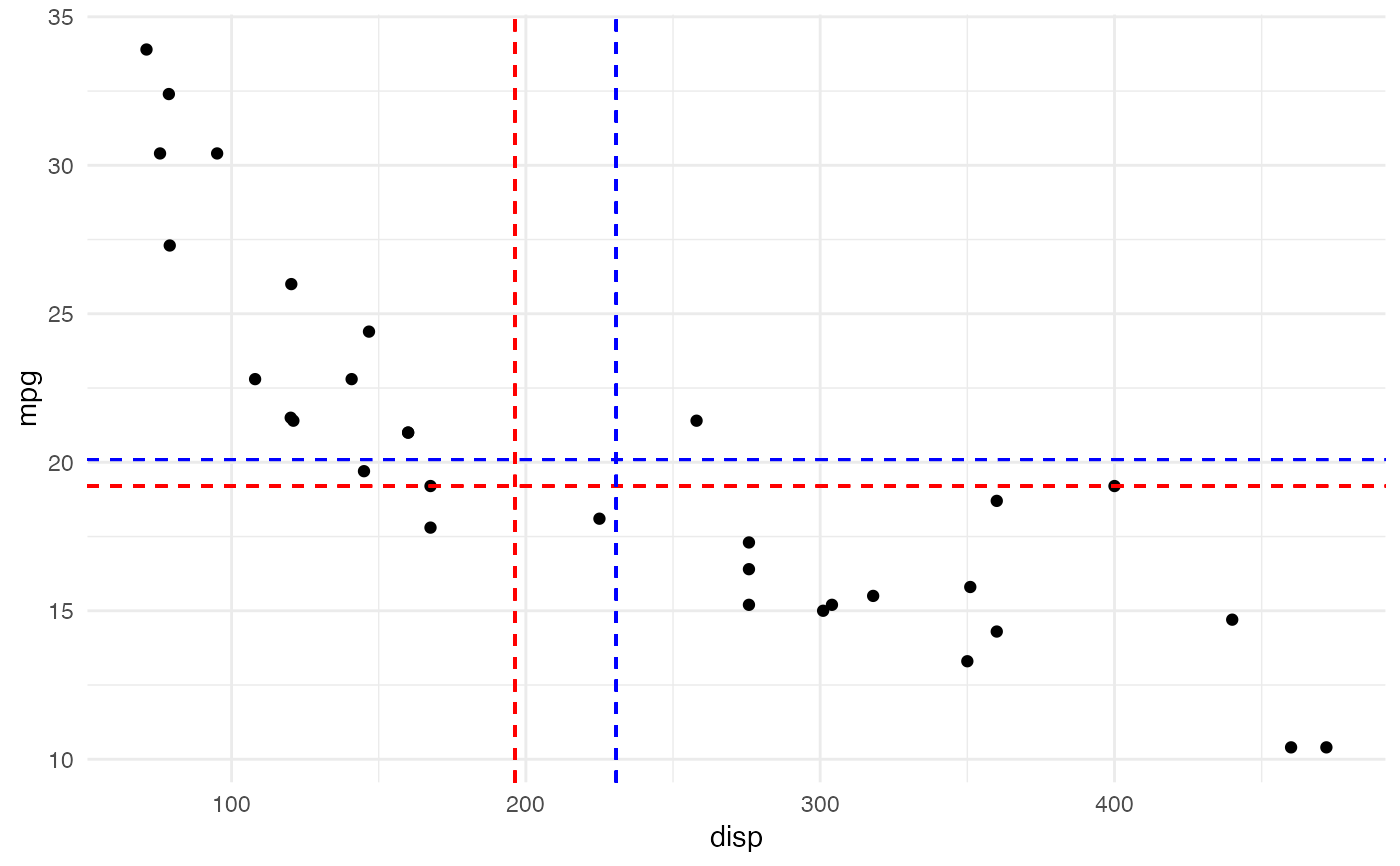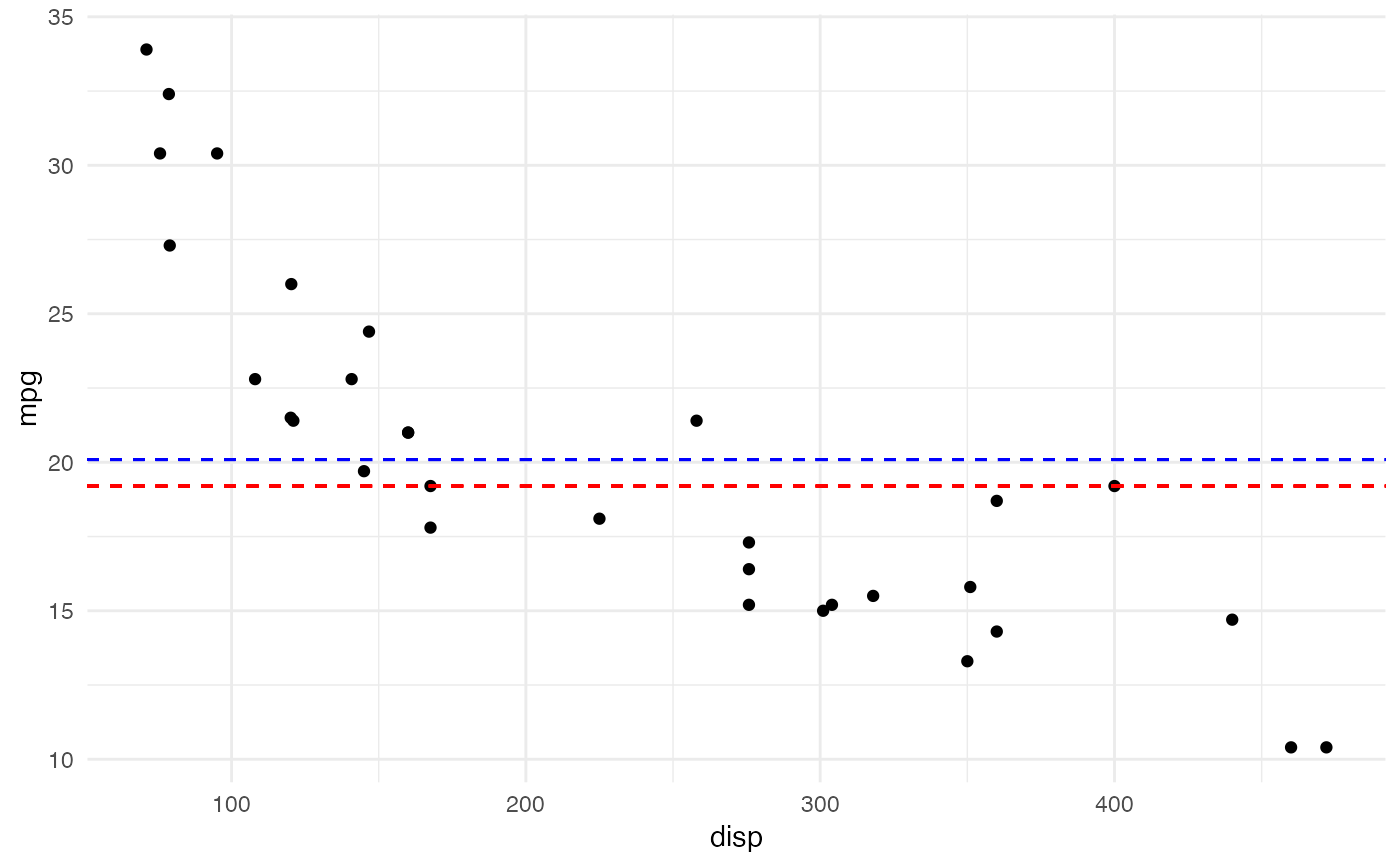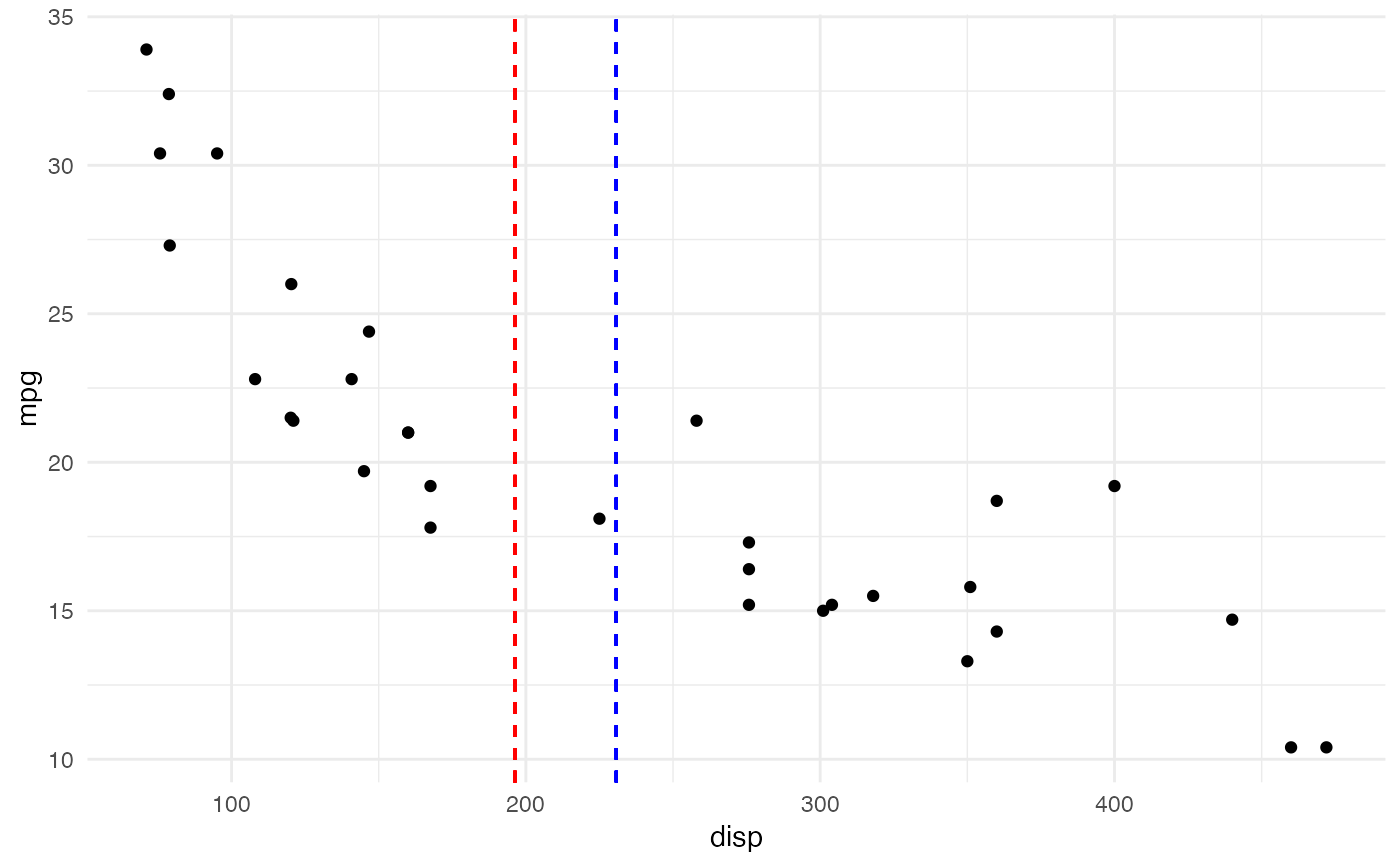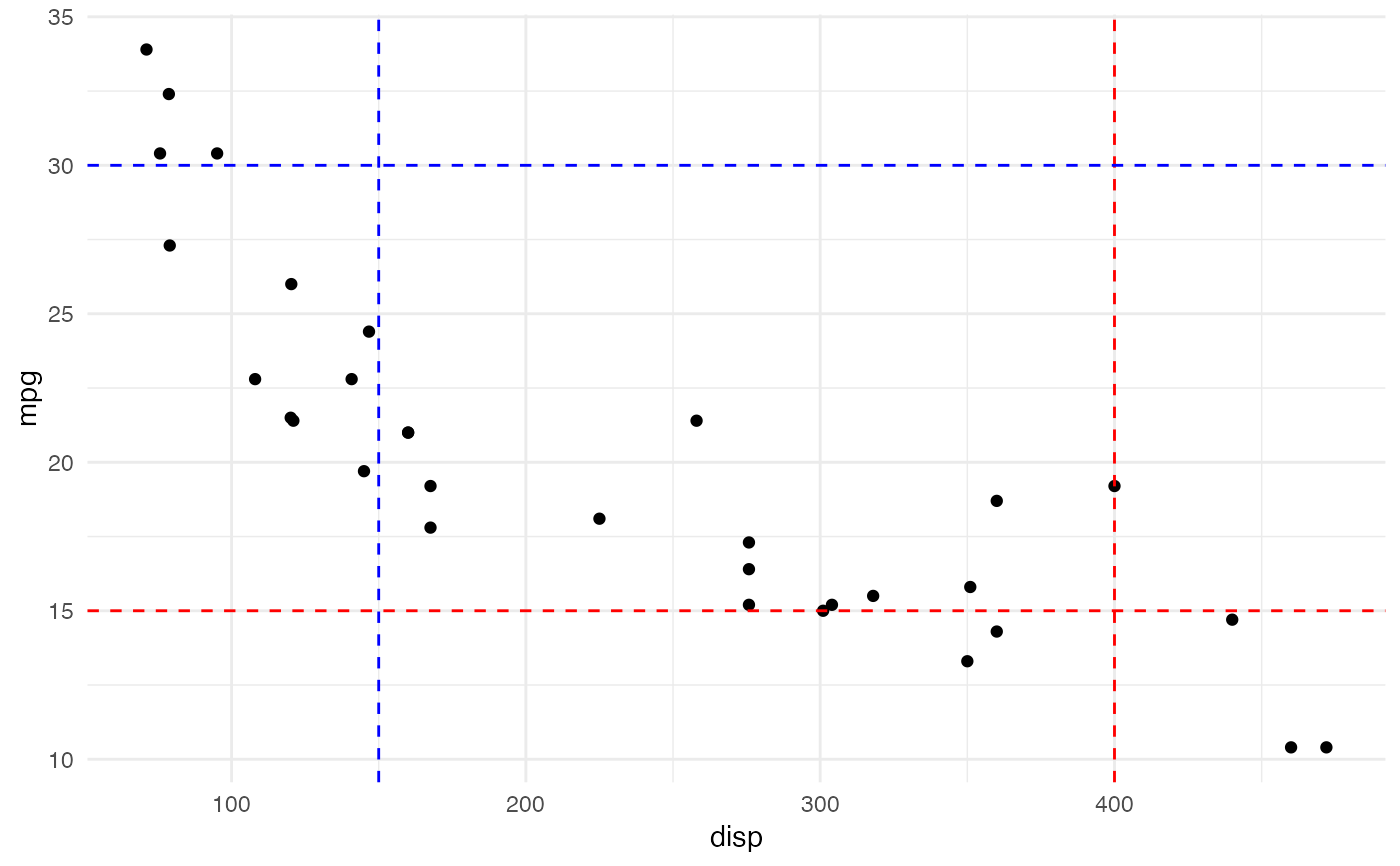These geoms can be used to draw horizontal or vertical reference lines in a ggplot. They use the data in the aesthetics v_var and h_var to compute their median or mean and draw the as a line.

geom_mean_lines(
mapping = NULL,
data = NULL,
...,
na.rm = FALSE,
show.legend = NA,
inherit.aes = TRUE
)

geom_median_lines(
mapping = NULL,
data = NULL,
...,
na.rm = FALSE,
show.legend = NA,
inherit.aes = TRUE
)

## Arguments

mapping Set of aesthetic mappings created by aes() or aes_(). The data to be displayed in this layer. There are three options: If NULL, the default, the data is inherited from the plot data as specified in the call to ggplot(). A data.frame, or other object, will override the plot data. All objects will be fortified to produce a data frame. See fortify() for which variables will be created. A function will be called with a single argument, the plot data. The return value must be a data.frame, and will be used as the layer data. A function can be created from a formula (e.g. ~ head(.x, 10)). Other arguments passed on to layer(). These are often aesthetics, used to set an aesthetic to a fixed value, like colour = "red" or size = 3. They may also be parameters to the paired geom/stat. If FALSE, the default, missing values are removed with a warning. If TRUE, missing values are silently removed. logical. Should this layer be included in the legends? NA, the default, includes if any aesthetics are mapped. FALSE never includes, and TRUE always includes. It can also be a named logical vector to finely select the aesthetics to display. If FALSE, overrides the default aesthetics, rather than combining with them. This is most useful for helper functions that define both data and aesthetics and shouldn't inherit behaviour from the default plot specification.

## Aesthetics

geom_median_lines() and geom_mean_lines() understand the following aesthetics (at least one of the bold aesthetics is required):

• v_var - The variable for which to compute the median/mean that is drawn as vertical line.

• h_var - TThe variable for which to compute the median/mean that is drawn as horizontal line.

• alpha = NA - The alpha channel, i.e. transparency level, as a numerical value between 0 and 1.

• color = "red" - The color of the drawn lines.

• linetype = 2 - The linetype of the drawn lines.

• size = 0.5 - The size of the drawn lines.

The underlying ggplot2 geoms geom_hline() and geom_vline()

## Examples

library(cfbplotR)
library(ggplot2)

# inherit top level aesthetics
ggplot(mtcars, aes(x = disp, y = mpg, h_var = mpg, v_var = disp)) +
geom_point() +
geom_median_lines() +
geom_mean_lines(color = "blue") +
theme_minimal()# draw horizontal line only
ggplot(mtcars, aes(x = disp, y = mpg, h_var = mpg)) +
geom_point() +
geom_median_lines() +
geom_mean_lines(color = "blue") +
theme_minimal()# draw vertical line only
ggplot(mtcars, aes(x = disp, y = mpg, v_var = disp)) +
geom_point() +
geom_median_lines() +
geom_mean_lines(color = "blue") +
theme_minimal()# choose your own value
ggplot(mtcars, aes(x = disp, y = mpg)) +
geom_point() +
geom_median_lines(v_var = 400, h_var = 15) +
geom_mean_lines(v_var = 150, h_var = 30, color = "blue") +
theme_minimal()